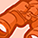# QlikView App Dev

Discussion Board for collaboration related to QlikView App Development.

Announcements
Now Live: Qlik Sense SaaS Simplified Authoring – Analytics Creation for Everyone: READ DETAILS
cancel
Showing results for
Did you mean:Not applicable

## Cumulative Percentages in a Chart

I have a chart with an expression of SUM(x) / SUM(y) that gives me results as percentages.

If I turn on "Full Accumulation", it sums the percentages.

What I would like is for each SUM() in my expression to sum the values from the preceding data points.

Example Data:

Series DimensionTime DimensionAnother DimensionXY
A1a12
A1b14
A2a23
A2b33
A3a34
A3b23
B1a23
B1b12
B2a34
B2b11
B3a34
B3b22

Which gives the following results:

Series DimensionTime DimensionPercentage
Cumulative
A1(1 + 1) / (1 + 4)(1 + 1) / (1 + 4)
A2(2 + 3) / (3 + 3)(1 + 1 + 2 + 3) / (1 + 4 + 3 + 3)
A3(3 + 2) / (4 + 3)(1 + 1 + 2 + 3 + 3 + 2) / (1 + 4 + 3 + 3 + 4 + 3)
B1(2 + 1) / (3 + 2)(2 + 1) / (3 + 2)
B2(3 + 1) / (4 + 1)(2 + 1 + 3 + 1) / (3 + 2 + 4 + 1)
B3(3 + 2) / (4 + 2)(2 + 1 + 3 + 1 + 3 + 2) / (3 + 2 + 4 + 1 + 4 + 2)

Is there a standard way to accomplish this in QlikView without doubling the number of fields in the data?  (field1 AND cumulativeField1, etc, etc)

Cheers,

Mat.

1 Solution

Accepted SolutionsNot applicable
Author

The problem is when I use a line chart.  I've updated your file to demonstrate...

10 RepliesNot applicable
Author

Hi,

You could probably use Chart Inter Record functions. Help page has some examples on this.Specialist

Hi,

As Hoekstra said, use inter record functions, in your case something like :

above(sum(X),0,rowno()) / above(sum(Y),0,rowno())

Regards,

VincentMVP

As Robert and Vincent both mentioned, chart inter records will do, I would just add a rangesum to Vincents expression to get a sum of the appropriate row values:

=rangesum(above(sum(X),0,rowno())) / rangesum(above(sum(Y),0,rowno()))

Regards,

StefanNot applicable
Author

Thanks.

This is almost there.  There are very stange things happening where I have two dimensions on the chart (Time and Series).

It appears to be mixing up the data between the different series.

But I'm making progress now.  I thinkMVP

So what is happening? Please note that the order of dimensions and the pivoting if using pivot table chart is affecting the results or the need to change above e.g. in before.

Please see above for a sample.

Regards,

StefanNot applicable
Author

The problem is when I use a line chart.  I've updated your file to demonstrate...MVP

Yes, you changed the order of dimensions, that's quite important for the column segments where the rangesum will sum the values in.

You could use

=aggr(RANGESUM(ABOVE(SUM(X), 0, ROWNO())) / RANGESUM(ABOVE(SUM(Y), 0, ROWNO())), [Series Dimension],[Time Dimension])

to correct this.

But then you might have a problem with the line chart (QV Line chart is not very clever done IMHO). I changed it to bar chart, that looks fine to me.

Regards,

Stefan

edit: my mistake, changing back to line chart should work also...Not applicable
Author

You sir, are a super massive exploding ball of hydrogen.

A star.

I can see that I have alot of reading to do regarding these functions.

Thanks,

Mat.Contributor II

Great post. I have come to a problem though. As soon as I add set analysis in the sum it stops working. Any idea why this could be happening?

=aggr(RANGESUM(ABOVE(SUM({<[Hit Decision]={'control-group'}>}[Transaction Counter]*[Net Reporting Amount Gross]), 0, ROWNO()))

,[Visit Date])